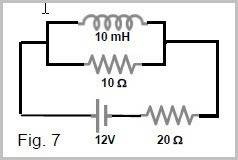# Find the rate of change of the current in the inductor

machinarium

## Homework Statement

For the circuit shown in Fig. 7, what is the rate of change of the current
in the inductor when the current in the battery is 0.50 A?
(1) 600 A/s (2) 400 A/s (3) 200 A/s (4) 800 A/s (5) 500 A/s## Homework Equations

i(t)=Imax(1-e-tR/L)
induced emf = L (dI/dt)

## The Attempt at a Solution

I didn't fully understand the problem. I don't understand why the current in the battery was given? What would I use that value for?

Please explain to me what I need to know to find out the rate of change of the current. Thank you very much.

#### Attachments

Homework Helper
Think: How is the voltage across the inductor related to the rate of change of current?

ehild

machinarium
Can you explain to me the whole problem? Please don't ask such a question, I was already very confused.

Thank you.

Homework Helper
The current through the battery is the same as through the 20 ohm resistor. The voltage across the inductor is the same as across the 10 ohm resistor, as they are connected in parallel. Can you find this voltage?

ehild

Mentor
I didn't fully understand the problem. I don't understand why the current in the battery was given? What would I use that value for?

Please explain to me what I need to know to find out the rate of change of the current. Thank you very much.

The current through the inductor will be changing with time. As a result, the total current being drawn from the battery will also change with time. By asking what the rate of change of the current through the inductor will be when the current through the battery is a given amount, it is equivalent to asking for the rate of change of the inductor current at a specific moment in time.

machinarium
Ah, I remembered it. Thank you for your commitment, ehild and gneill

The potential through the inductor is 12 – (20 ohm X 0.5 A) = 2 V
Then dI/dt=2V/10mH=200 A/s
Is that correct?

Mentor

You might refer to the potential across the inductor, rather than through the inductor; Potentials are "across", currents are "through".

Homework Helper
You might refer to the potential across the inductor, rather than through the inductor; Potentials are "across", currents are "through".

Potential is defined at a point. Potential difference or voltage is across a resistor or coil, or between two points.

ehild

Mentor
Potential is defined at a point. Potential difference or voltage is across a resistor or coil, or between two points.

ehild

"Across" implies two points are involved. "potential across" is sloppy shorthand for potential difference across. mea culpa.